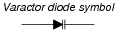# Special Diodes

## Discrete Semiconductor Devices and Circuits

• #### Question 1

 Don’t just sit there! Build something!!

Learning to mathematically analyze circuits requires much study and practice. Typically, students practice by working through lots of sample problems and checking their answers against those provided by the textbook or the instructor. While this is good, there is a much better way.

You will learn much more by actually building and analyzing real circuits, letting your test equipment provide the änswers” instead of a book or another person. For successful circuit-building exercises, follow these steps:

1. Carefully measure and record all component values prior to circuit construction, choosing resistor values high enough to make damage to any active components unlikely.
2. Draw the schematic diagram for the circuit to be analyzed.
3. Carefully build this circuit on a breadboard or other convenient medium.
4. Check the accuracy of the circuit’s construction, following each wire to each connection point, and verifying these elements one-by-one on the diagram.
5. Mathematically analyze the circuit, solving for all voltage and current values.
6. Carefully measure all voltages and currents, to verify the accuracy of your analysis.
7. If there are any substantial errors (greater than a few percent), carefully check your circuit’s construction against the diagram, then carefully re-calculate the values and re-measure.

When students are first learning about semiconductor devices, and are most likely to damage them by making improper connections in their circuits, I recommend they experiment with large, high-wattage components (1N4001 rectifying diodes, TO-220 or TO-3 case power transistors, etc.), and using dry-cell battery power sources rather than a benchtop power supply. This decreases the likelihood of component damage.

As usual, avoid very high and very low resistor values, to avoid measurement errors caused by meter “loading” (on the high end) and to avoid transistor burnout (on the low end). I recommend resistors between 1 kΩ and 100 kΩ.

One way you can save time and reduce the possibility of error is to begin with a very simple circuit and incrementally add components to increase its complexity after each analysis, rather than building a whole new circuit for each practice problem. Another time-saving technique is to re-use the same components in a variety of different circuit configurations. This way, you won’t have to measure any component’s value more than once.

• #### Question 2

The characteristically colored glow from a gas-discharge electric light is the result of energy emitted by electrons in the gas atoms as they fall from high-level “excited” states back to their natural (“ground”) states. As a general rule of electron behavior, they must absorb energy from an external source to leap into a higher level, and they release that energy upon returning to their original level.

Given the existence of this phenomenon, what do you suspect might be occurring inside a PN junction as it conducts an electric current?

• #### Question 3

Explain the operating principle of a photovoltaic cell, otherwise known as a “solar cell.” What happens within these devices to convert sunlight directly into electricity?

• #### Question 4

The nonconducting depletion region of a PN junction forms a parasitic capacitance between the P and the N semiconductor region. Does the capacitance increase or decrease as a greater reverse-bias voltage is applied to the PN junction? Explain your answer.

• #### Question 5

Explain what a Schottky diode is, and how it differs in construction and in function from a normal semiconductor PN junction diode.

• #### Question 6

Draw the schematic symbol for a Schottky diode, and give some examples of typical applications for it.

• #### Question 7

There is a special type of diode called a varactor, which is used to create a voltage-dependent capacitance. This function is often used in electronic radio tuner circuits:The voltage-dependent capacitance of this diode is given by the following equation:

 $$C_j = \frac{C_o}{\sqrt{2V+1}}$$

Where,

CJ = Junction capacitance

Co = Junction capacitance with no applied voltage

V = Applied reverse junction voltage

Based on this equation, would you say that capacitance is directly or inversely related to the applied reverse-bias voltage of a varactor diode? Based on what you know of diode theory, explain why this makes sense.